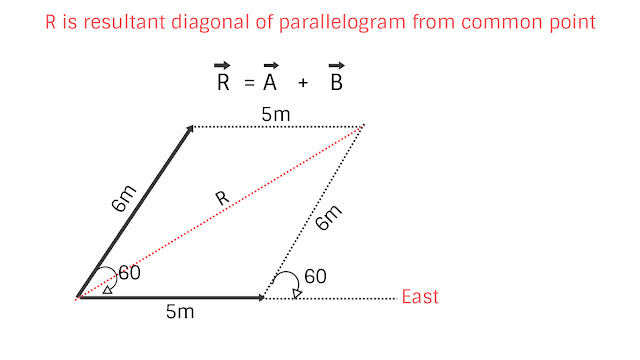Vector important operation concept

This topic is very very important for physics concept better understand as we have already discussed in previous two post for vector concept in more details your can refer we know that vector is those quantities which has magnitude as well as direction in this topic we will learn how vector operation is perform and why vector is one separate topic.
see below picture we will analyse why vector is separate topic ?Vector important operation conceptsuppose i have 4kg rice and you have 6kg rice if we add this we will get (4kg +6kg )=10kg rice no doubt, now take other example  you moved 4m in east and 3m in north, understand here 4m is displacement and 3m is also displacement if i asked how far you are from starting point now your answer will be 5m and 5 is also displacement  how this addition comes 4m+3m = 5m, take one more example you move 3m in east this is displacement and take a sharp turn 180⁰and move 4m west 4m is also displacement now i ask how far you are from your starting point your answer will be 1m this 1m is also displacement again how this addition come 3m+4m = 1m this is the operation of vector addition and that is why vector is one separate topic this operation is totally different from scalar operation, now take a third operation you move 4m east this is displacement then move 3m at an angle of 60⁰ with east, 3m is also displacement now i ask how far you are from your starting point now it will not be  4m+3m = 7m nor 5m because it is not at right angle now so it will not be 5m here we have to take component method of addition then we will get the result or resultant so this operation is totally different from scalar operation we can’t add vector like rice, mass, time these all are scalar quantities so we need a separate topic for vector operation and it is important because all important quantities like displacement, velocity, acceleration, force, torque, electric field, magnetic field and many more all are vector quantities and will follow this rule for addition and other operation hence it is very important to understand vector algebra operation. Vector Addition: now there are three method for vector addition.1  Head – Tail method  (basic method )2  Parallelogram law method 3  Triangle law method now for any vector addition we have to apply any one method to add vector according to situation which one can apply easily so lets start one by one method.Head – Tail method : in this method suppose you want to add more than two vector it can be 3,5, 10 or more vector just add like this “join tail of next vector with head of previous vector and continue joining till last vector” and whenever you want answer or resultant then join tail of first vector with head of last vector here in vector answer is resultant.This is basic only at right angle vector can be added through this method if two vector are at some angle this method is not applicable to find out resultant as above Red color vector is resultant so for this type of vector we use parallelogram method.Parallelogram law method: This law is applicable to add any type of two vector “Join two vector from tail to tail as two adjacent side of a parallelogram complete parallelogram” Suppose one vector A is 5m east and second vector B is 6m with 60⁰ East.where R is resultant and its value is . R² = A²+B²+2ABCos?  where  ? is angle between two vector A and B this formula can be drive we will drive this formula in component vector section for now just remember now put all value A = 5, B =6 in the above equation we will get R² = 5²+6²+2*5*6cos60 = 25+36+60*1/2 =  91 R =√91 take R value only positive since R resultant is a vector hence it has direction also R direction from A let is ? angle then  tan? = Bsin?/(A+Bcos?) now we will see derivation here .Vector tail to tail join MP and MN Now here see ∠NMP = ? and ∠OMP = ? since MPON is a parallelogram so MN = PO and ∠NMP = ∠OPQ  = ? now take in triangle OPQ we can write cos? = PQ/PO = PQ/B hence  PQ = Bcos? in same triangle  sin? = OQ/OP = OQ/B  OQ = Bsin?  Now see in tangle OMQ this is right angle triangle hence we can write OM²  = MQ² + QO² also we can write as  OM² = (MP+PQ)² +QO² now put value MP = A, PQ = Bcos? and QO = Bsin? OM = R R²  = (A+Bcos?)² +(Bsin?)²  = A²+B²cos?²+2ABcos? +B²sin?² now simplify we will get  R² = A²+B²+2ABCos?. Now for direction of resultant in ∆OMQ we can write  tan? = OQ/MQ =           tan? = Bsin?/(A+Bcos?)  important point for vector angle always take angle between two vector by joining tail to tail or head to head always student confused about angle determination so you must remember this point now we can do some simple basic problem on this topic. Q Two vector of equal magnitude are added to give resultant which is of same magnitude as the two vectors find the angle between two vector. Ans First read question carefully you will get condition from question here two vector A, B and resultant are equal from question hence A= B = R Now use formula for resultant R. R² = A²+B²+2ABCos?  let R = A= B =x now put  x²  = x²+x²+2x²Cos?  now simplify we will get  Cos? = -1/2  ?  = 120⁰  value of x#0 now you can solve any type of problem using this formula formula in next post we will continue more about vector operation thanks for reading. Vector important operation concept  dated 3-Jun-2018